# Chapter 11 Chemical Reactions Types of Chemical Reactions

• Slides: 34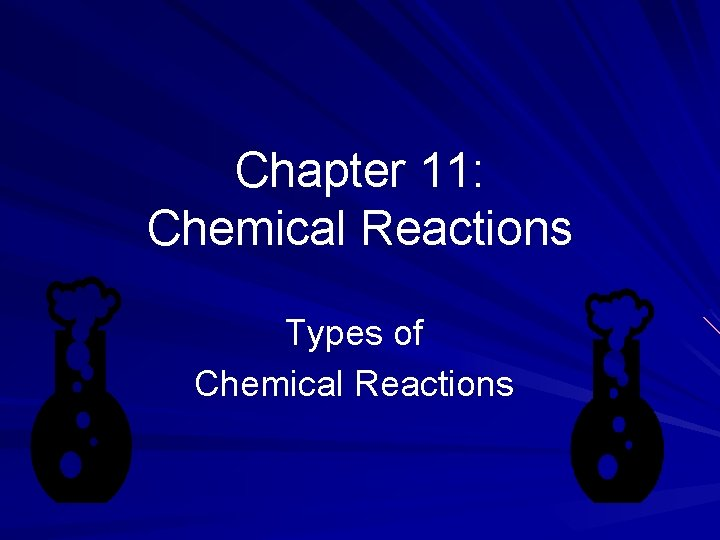Chapter 11: Chemical Reactions Types of Chemical ReactionsLearning Targets You will recognize the type of chemical reaction taking place: oxidation-reduction – synthesis, decomposition, single replacement, combustion; double replacement – precipitation, acid base reactions, gas forming reactions. You will predict the products of a chemical reaction when given the reactants and write a balanced chemical equation. You will determine the solubility of a substance. You will determine the activity of a metal or halogen when predicting the products of a chemical reaction.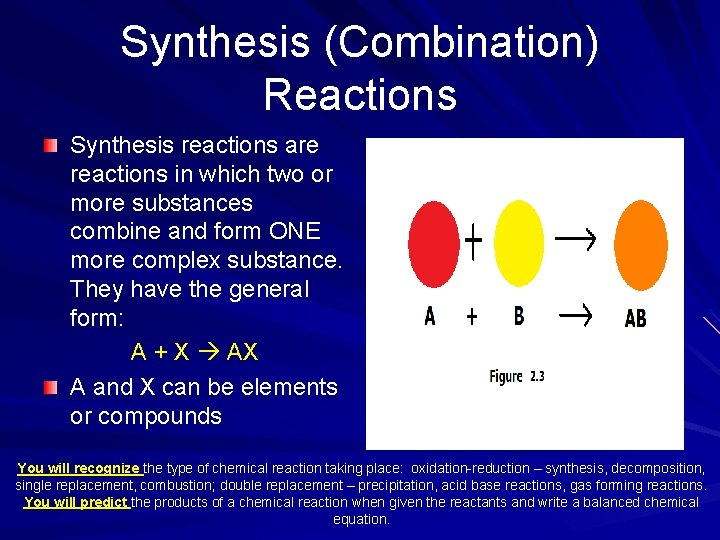Synthesis (Combination) Reactions Synthesis reactions are reactions in which two or more substances combine and form ONE more complex substance. They have the general form: A + X AX A and X can be elements or compounds You will recognize the type of chemical reaction taking place: oxidation-reduction – synthesis, decomposition, single replacement, combustion; double replacement – precipitation, acid base reactions, gas forming reactions. You will predict the products of a chemical reaction when given the reactants and write a balanced chemical equation.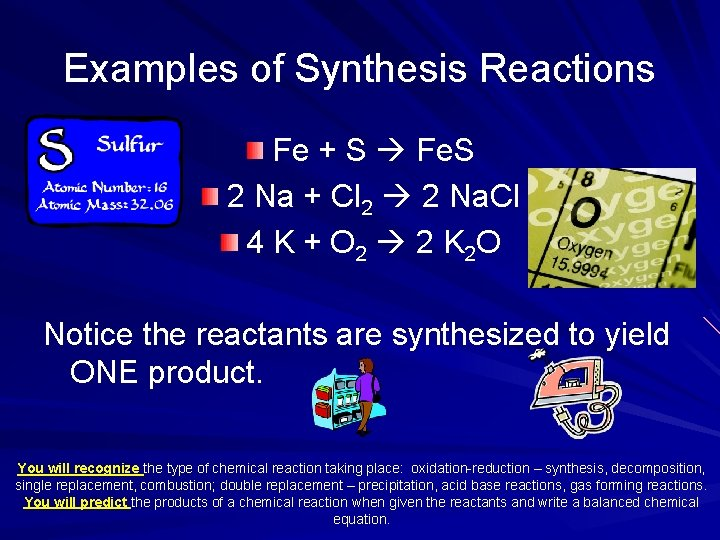Examples of Synthesis Reactions Fe + S Fe. S 2 Na + Cl 2 2 Na. Cl 4 K + O 2 2 K 2 O Notice the reactants are synthesized to yield ONE product. You will recognize the type of chemical reaction taking place: oxidation-reduction – synthesis, decomposition, single replacement, combustion; double replacement – precipitation, acid base reactions, gas forming reactions. You will predict the products of a chemical reaction when given the reactants and write a balanced chemical equation.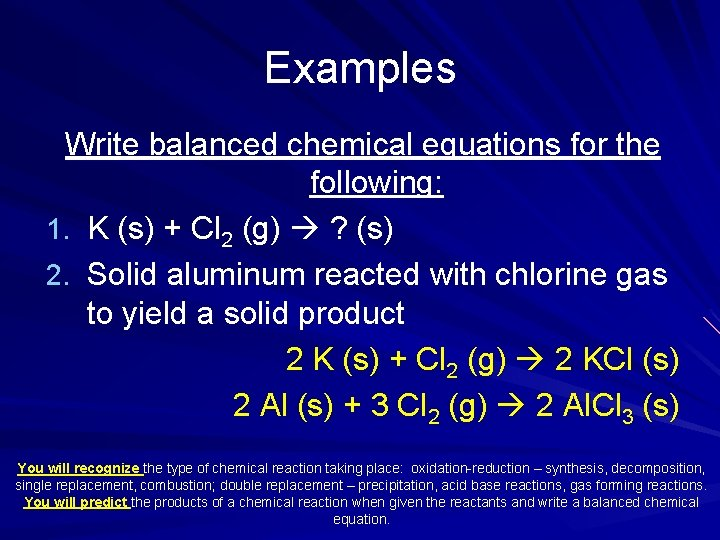Examples Write balanced chemical equations for the following: 1. K (s) + Cl 2 (g) ? (s) 2. Solid aluminum reacted with chlorine gas to yield a solid product 2 K (s) + Cl 2 (g) 2 KCl (s) 2 Al (s) + 3 Cl 2 (g) 2 Al. Cl 3 (s) You will recognize the type of chemical reaction taking place: oxidation-reduction – synthesis, decomposition, single replacement, combustion; double replacement – precipitation, acid base reactions, gas forming reactions. You will predict the products of a chemical reaction when given the reactants and write a balanced chemical equation.Try on your own Write balanced chemical equations for the following: 1. Solid cesium reacted with liquid bromine to yield a solid product 2. Mg (s) + O 2 (g) ? (s) 2 Cs (s) + Br 2 (l) 2 Cs. Br (s) 2 Mg (s) + O 2 (g) 2 Mg. O (s) You will recognize the type of chemical reaction taking place: oxidation-reduction – synthesis, decomposition, single replacement, combustion; double replacement – precipitation, acid base reactions, gas forming reactions. You will predict the products of a chemical reaction when given the reactants and write a balanced chemical equation.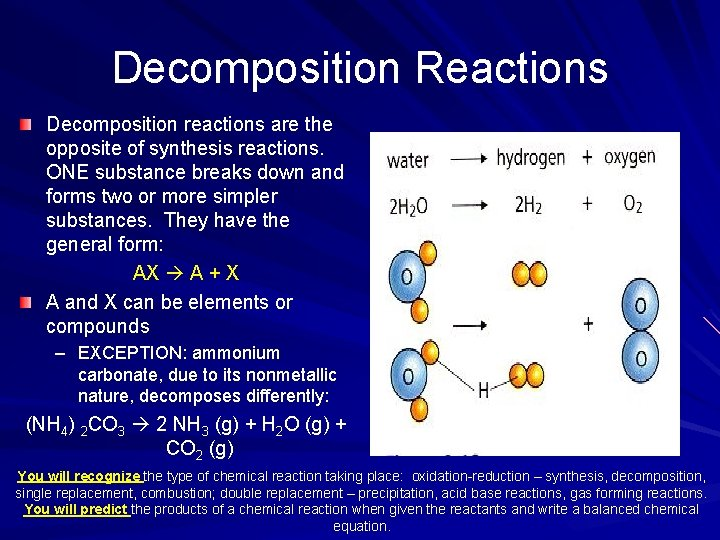Decomposition Reactions Decomposition reactions are the opposite of synthesis reactions. ONE substance breaks down and forms two or more simpler substances. They have the general form: AX A + X A and X can be elements or compounds – EXCEPTION: ammonium carbonate, due to its nonmetallic nature, decomposes differently: (NH 4) 2 CO 3 2 NH 3 (g) + H 2 O (g) + CO 2 (g) You will recognize the type of chemical reaction taking place: oxidation-reduction – synthesis, decomposition, single replacement, combustion; double replacement – precipitation, acid base reactions, gas forming reactions. You will predict the products of a chemical reaction when given the reactants and write a balanced chemical equation.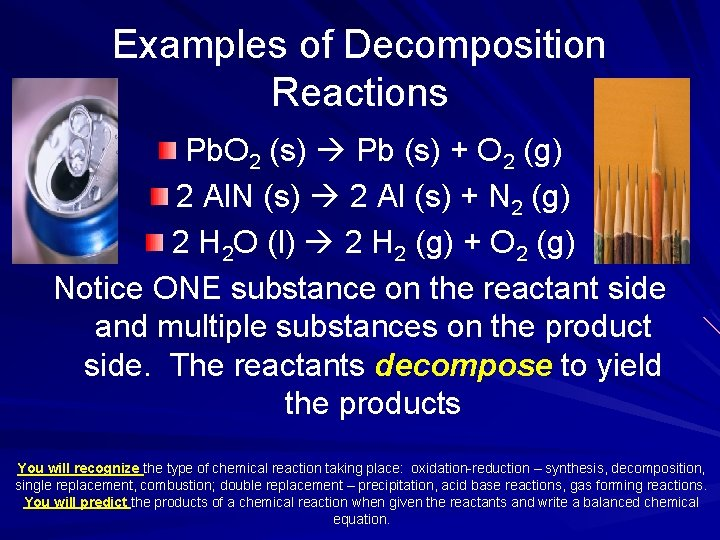Examples of Decomposition Reactions Pb. O 2 (s) Pb (s) + O 2 (g) 2 Al. N (s) 2 Al (s) + N 2 (g) 2 H 2 O (l) 2 H 2 (g) + O 2 (g) Notice ONE substance on the reactant side and multiple substances on the product side. The reactants decompose to yield the products You will recognize the type of chemical reaction taking place: oxidation-reduction – synthesis, decomposition, single replacement, combustion; double replacement – precipitation, acid base reactions, gas forming reactions. You will predict the products of a chemical reaction when given the reactants and write a balanced chemical equation.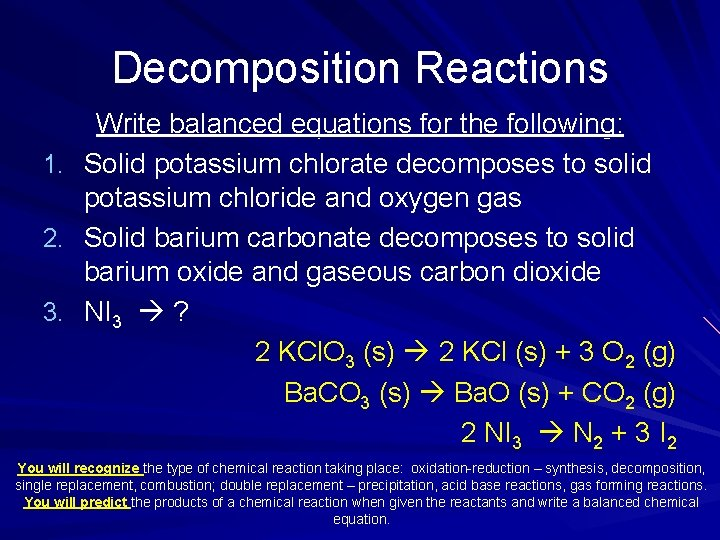Decomposition Reactions 1. 2. 3. Write balanced equations for the following: Solid potassium chlorate decomposes to solid potassium chloride and oxygen gas Solid barium carbonate decomposes to solid barium oxide and gaseous carbon dioxide NI 3 ? 2 KCl. O 3 (s) 2 KCl (s) + 3 O 2 (g) Ba. CO 3 (s) Ba. O (s) + CO 2 (g) 2 NI 3 N 2 + 3 I 2 You will recognize the type of chemical reaction taking place: oxidation-reduction – synthesis, decomposition, single replacement, combustion; double replacement – precipitation, acid base reactions, gas forming reactions. You will predict the products of a chemical reaction when given the reactants and write a balanced chemical equation.Let’s check in … 1. Write the formula for the binary compound that decomposes to the products H 2 and Br 2? 2. Complete and balance this decomposition reaction. HI 3. Write and balance the equation for the formation of magnesium nitride (Mg 3 N 2) from its elements. You will recognize the type of chemical reaction taking place: oxidation-reduction – synthesis, decomposition, single replacement, combustion; double replacement – precipitation, acid base reactions, gas forming reactions. You will predict the products of a chemical reaction when given the reactants and write a balanced chemical equation.How did you do? 1. Write the formula for the binary compound that decomposes to the products H 2 and Br 2? HBr (g) 2. Complete and balance this decomposition reaction. 2 HI H 2 (g) + I 2 (g) 3. Write and balance the equation for the formation of magnesium nitride (Mg 3 N 2) from its elements. 3 Mg (s) + N 2 (g) Mg 3 N 2 (s) You will recognize the type of chemical reaction taking place: oxidation-reduction – synthesis, decomposition, single replacement, combustion; double replacement – precipitation, acid base reactions, gas forming reactions. You will predict the products of a chemical reaction when given the reactants and write a balanced chemical equation.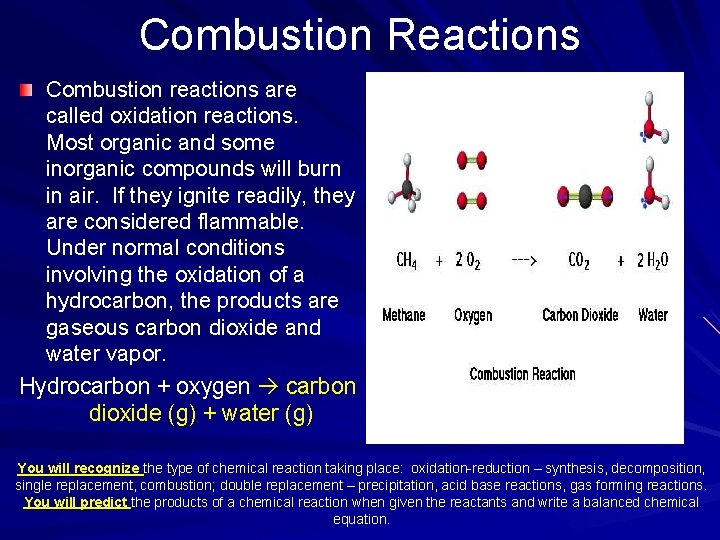Combustion Reactions Combustion reactions are called oxidation reactions. Most organic and some inorganic compounds will burn in air. If they ignite readily, they are considered flammable. Under normal conditions involving the oxidation of a hydrocarbon, the products are gaseous carbon dioxide and water vapor. Hydrocarbon + oxygen carbon dioxide (g) + water (g) You will recognize the type of chemical reaction taking place: oxidation-reduction – synthesis, decomposition, single replacement, combustion; double replacement – precipitation, acid base reactions, gas forming reactions. You will predict the products of a chemical reaction when given the reactants and write a balanced chemical equation.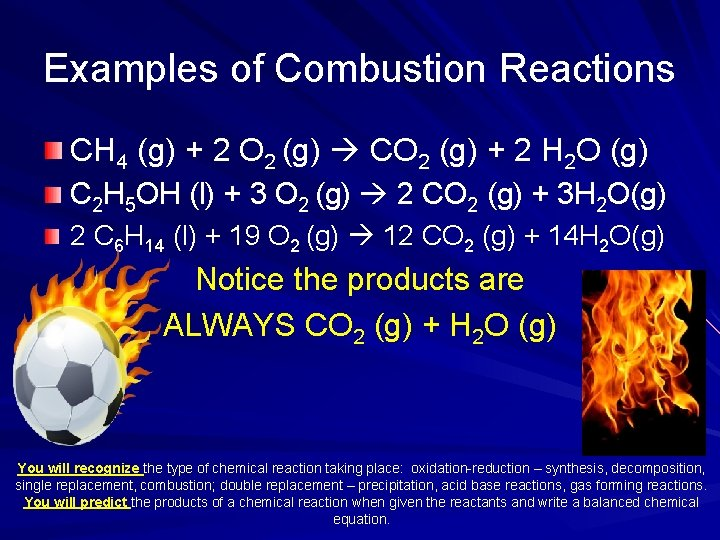Examples of Combustion Reactions CH 4 (g) + 2 O 2 (g) CO 2 (g) + 2 H 2 O (g) C 2 H 5 OH (l) + 3 O 2 (g) 2 CO 2 (g) + 3 H 2 O(g) 2 C 6 H 14 (l) + 19 O 2 (g) 12 CO 2 (g) + 14 H 2 O(g) Notice the products are ALWAYS CO 2 (g) + H 2 O (g) You will recognize the type of chemical reaction taking place: oxidation-reduction – synthesis, decomposition, single replacement, combustion; double replacement – precipitation, acid base reactions, gas forming reactions. You will predict the products of a chemical reaction when given the reactants and write a balanced chemical equation.Combustion Reactions 1. 2. 3. Write balanced equations for the following reactions: Butane (C 4 H 10) gas burns in oxygen C 2 H 6 (l) + O 2 (g) C 2 H 6 O (l) + O 2 (g) 2 C 4 H 10 (g) + 13 O 2 (g) 8 CO 2 (g) + 10 H 2 O (g) 2 C 2 H 6 (l) + 7 O 2 (g) 4 CO 2 (g) + 6 H 2 O (g) C 2 H 6 O (l) + 3 O 2 (g) 2 CO 2 (g) + 3 H 2 O (g) You will recognize the type of chemical reaction taking place: oxidation-reduction – synthesis, decomposition, single replacement, combustion; double replacement – precipitation, acid base reactions, gas forming reactions. You will predict the products of a chemical reaction when given the reactants and write a balanced chemical equation.Let’s check in … Write a balanced equation for the complete combustion of each compound. 1. Formaldehyde (CH 2 O (g)) 2. Heptane (C 7 H 16 (l)) 3. Benzene (C 6 H 6 (l)) You will recognize the type of chemical reaction taking place: oxidation-reduction – synthesis, decomposition, single replacement, combustion; double replacement – precipitation, acid base reactions, gas forming reactions. You will predict the products of a chemical reaction when given the reactants and write a balanced chemical equation.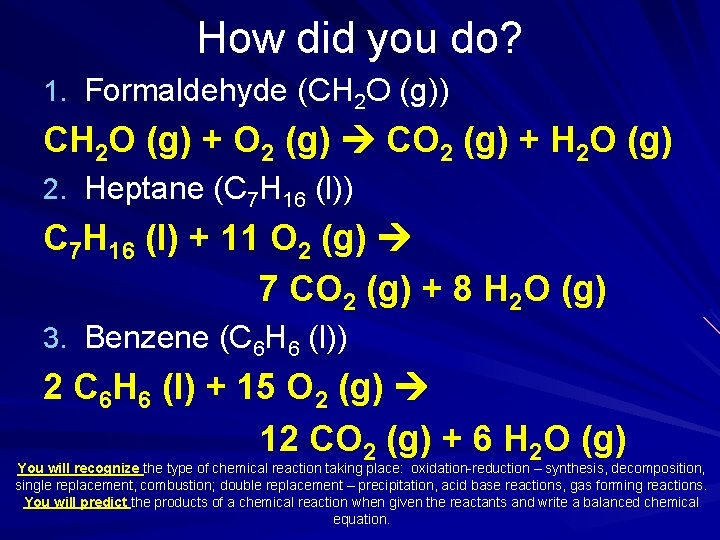How did you do? 1. Formaldehyde (CH 2 O (g)) CH 2 O (g) + O 2 (g) CO 2 (g) + H 2 O (g) 2. Heptane (C 7 H 16 (l)) C 7 H 16 (l) + 11 O 2 (g) 7 CO 2 (g) + 8 H 2 O (g) 3. Benzene (C 6 H 6 (l)) 2 C 6 H 6 (l) + 15 O 2 (g) 12 CO 2 (g) + 6 H 2 O (g) You will recognize the type of chemical reaction taking place: oxidation-reduction – synthesis, decomposition, single replacement, combustion; double replacement – precipitation, acid base reactions, gas forming reactions. You will predict the products of a chemical reaction when given the reactants and write a balanced chemical equation.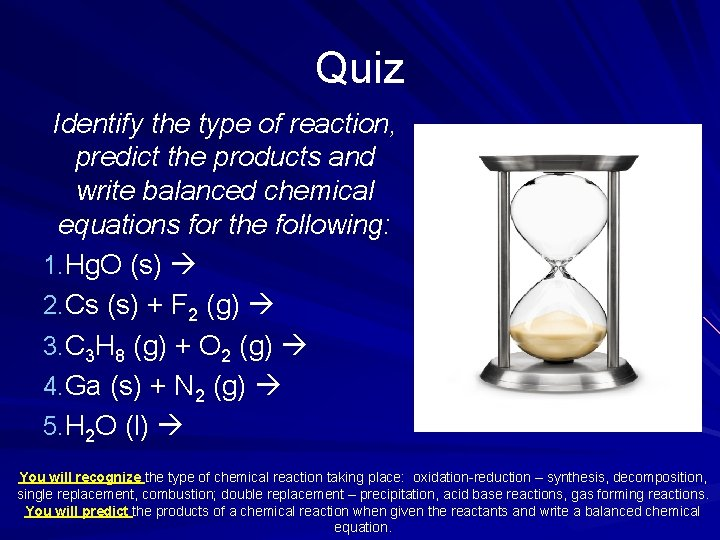Quiz Identify the type of reaction, predict the products and write balanced chemical equations for the following: 1. Hg. O (s) 2. Cs (s) + F 2 (g) 3. C 3 H 8 (g) + O 2 (g) 4. Ga (s) + N 2 (g) 5. H 2 O (l) You will recognize the type of chemical reaction taking place: oxidation-reduction – synthesis, decomposition, single replacement, combustion; double replacement – precipitation, acid base reactions, gas forming reactions. You will predict the products of a chemical reaction when given the reactants and write a balanced chemical equation.Answers 1. Decomposition - 2 Hg. O (s) 2 Hg + O 2 2. Synthesis - 2 Cs (s) + F 2 (g) 2 Cs. F 3. Combustion – C 3 H 8 (g) + 5 O 2 (g) 3 CO 2 (g) + 4 H 2 O (g) 4. Synthesis - 2 Ga (s) + N 2 (g) 2 Ga. N 5. Decomposition - 2 H 2 O (l) 2 H 2 + O 2 You will recognize the type of chemical reaction taking place: oxidation-reduction – synthesis, decomposition, single replacement, combustion; double replacement – precipitation, acid base reactions, gas forming reactions. You will predict the products of a chemical reaction when given the reactants and write a balanced chemical equation.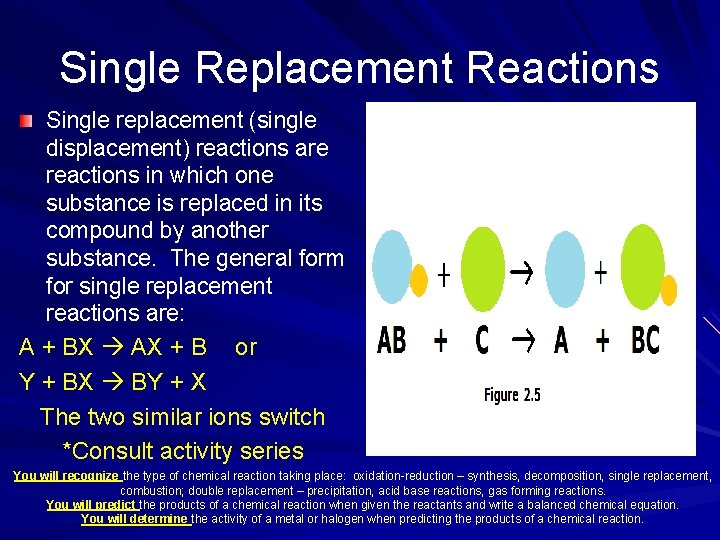Single Replacement Reactions Single replacement (single displacement) reactions are reactions in which one substance is replaced in its compound by another substance. The general form for single replacement reactions are: A + BX AX + B or Y + BX BY + X The two similar ions switch *Consult activity series You will recognize the type of chemical reaction taking place: oxidation-reduction – synthesis, decomposition, single replacement, combustion; double replacement – precipitation, acid base reactions, gas forming reactions. You will predict the products of a chemical reaction when given the reactants and write a balanced chemical equation. You will determine the activity of a metal or halogen when predicting the products of a chemical reaction.Examples of Single Replacement Reactions 1. Fe 2 O 3 (s) + 2 Al (s) Al 2 O 3 (s) + 2 Fe (s) 2. 2 HCl (aq) + Zn (s) H 2 (g) + Zn. Cl 2 (aq) 3. Zn (s) + Cu. SO 4 (aq) Zn. SO 4 (aq) +Cu(s) Notice the single element replaces the similar ion in the compound to produce a new element and new compound You will recognize the type of chemical reaction taking place: oxidation-reduction – synthesis, decomposition, single replacement, combustion; double replacement – precipitation, acid base reactions, gas forming reactions. You will predict the products of a chemical reaction when given the reactants and write a balanced chemical equation. You will determine the activity of a metal or halogen when predicting the products of a chemical reaction.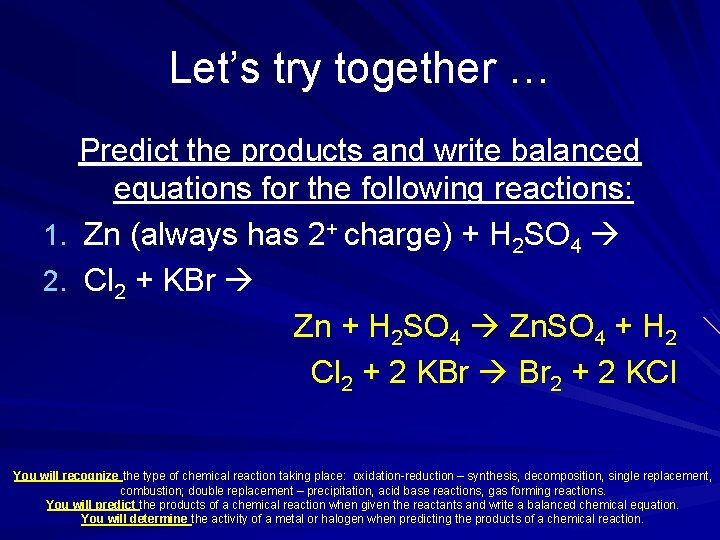Let’s try together … Predict the products and write balanced equations for the following reactions: 1. Zn (always has 2+ charge) + H 2 SO 4 2. Cl 2 + KBr Zn + H 2 SO 4 Zn. SO 4 + H 2 Cl 2 + 2 KBr Br 2 + 2 KCl You will recognize the type of chemical reaction taking place: oxidation-reduction – synthesis, decomposition, single replacement, combustion; double replacement – precipitation, acid base reactions, gas forming reactions. You will predict the products of a chemical reaction when given the reactants and write a balanced chemical equation. You will determine the activity of a metal or halogen when predicting the products of a chemical reaction.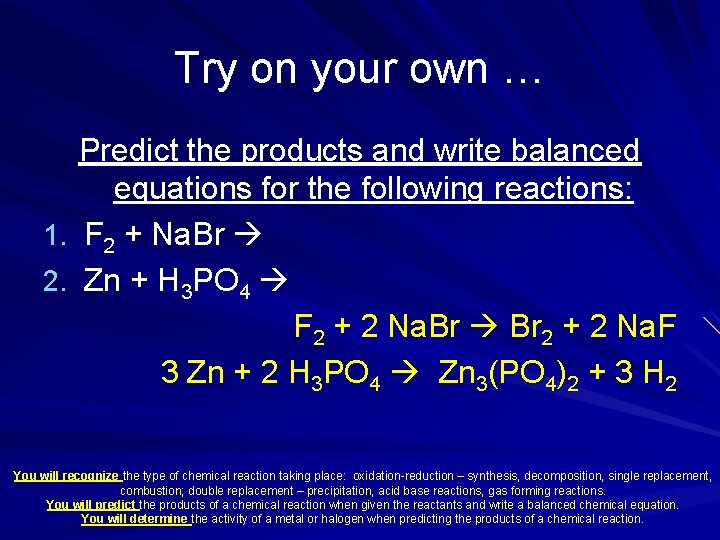Try on your own … Predict the products and write balanced equations for the following reactions: 1. F 2 + Na. Br 2. Zn + H 3 PO 4 F 2 + 2 Na. Br 2 + 2 Na. F 3 Zn + 2 H 3 PO 4 Zn 3(PO 4)2 + 3 H 2 You will recognize the type of chemical reaction taking place: oxidation-reduction – synthesis, decomposition, single replacement, combustion; double replacement – precipitation, acid base reactions, gas forming reactions. You will predict the products of a chemical reaction when given the reactants and write a balanced chemical equation. You will determine the activity of a metal or halogen when predicting the products of a chemical reaction.Let’s check in … Complete the equations for these singlereplacement reactions in aqueous solution. Balance each equation. Write “no reaction” if a reaction does not occur. 1. Fe (s) (iron has a +2 charge) + Pb(NO 3)2 (aq) 2. Cl 2 (aq) + Na. I (aq) 3. Ca (s) + H 2 O (l) 4. Zn (s) + H 2 SO 4 (aq) You will recognize the type of chemical reaction taking place: oxidation-reduction – synthesis, decomposition, single replacement, combustion; double replacement – precipitation, acid base reactions, gas forming reactions. You will predict the products of a chemical reaction when given the reactants and write a balanced chemical equation. You will determine the activity of a metal or halogen when predicting the products of a chemical reaction.How did you do? 1. Fe (s) + Pb(NO 3)2 (aq) Fe(NO 3)2 (aq) + Pb (s) 2. Cl 2 (aq) + 2 Na. I (aq) 2 Na. Cl (aq) + I 2 (aq) 3. Ca (s) + 2 H 2 O (l) Ca(OH)2 (aq) + H 2 (g) 4. Zn (s) + H 2 SO 4 (aq) Zn. SO 4 + H 2 You will recognize the type of chemical reaction taking place: oxidation-reduction – synthesis, decomposition, single replacement, combustion; double replacement – precipitation, acid base reactions, gas forming reactions. You will predict the products of a chemical reaction when given the reactants and write a balanced chemical equation. You will determine the activity of a metal or halogen when predicting the products of a chemical reaction.Double Replacement Reactions Ions in solutions combine and form a product. These reactions are also known as ionic reactions with the following form: AX (aq) + BY (aq) AY + BX In order for a reaction to have occurred the reactants must be soluble and a product must be formed. The product may be a solid, gas or liquid. CONSULT SOLUBILITY CHART! You will recognize the type of chemical reaction taking place: oxidation-reduction – synthesis, decomposition, single replacement, combustion; double replacement – precipitation, acid base reactions, gas forming reactions. You will predict the products of a chemical reaction when given the reactants and write a balanced chemical equation. You will determine the solubility of a substance.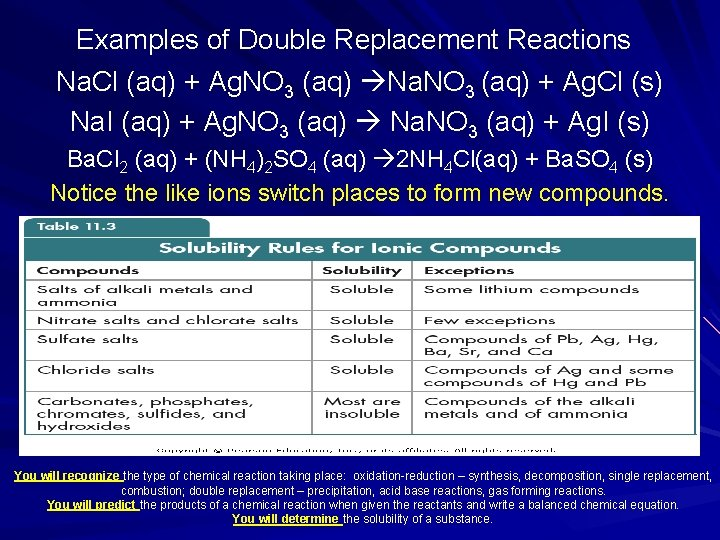Examples of Double Replacement Reactions Na. Cl (aq) + Ag. NO 3 (aq) Na. NO 3 (aq) + Ag. Cl (s) Na. I (aq) + Ag. NO 3 (aq) Na. NO 3 (aq) + Ag. I (s) Ba. Cl 2 (aq) + (NH 4)2 SO 4 (aq) 2 NH 4 Cl(aq) + Ba. SO 4 (s) Notice the like ions switch places to form new compounds. You will recognize the type of chemical reaction taking place: oxidation-reduction – synthesis, decomposition, single replacement, combustion; double replacement – precipitation, acid base reactions, gas forming reactions. You will predict the products of a chemical reaction when given the reactants and write a balanced chemical equation. You will determine the solubility of a substance.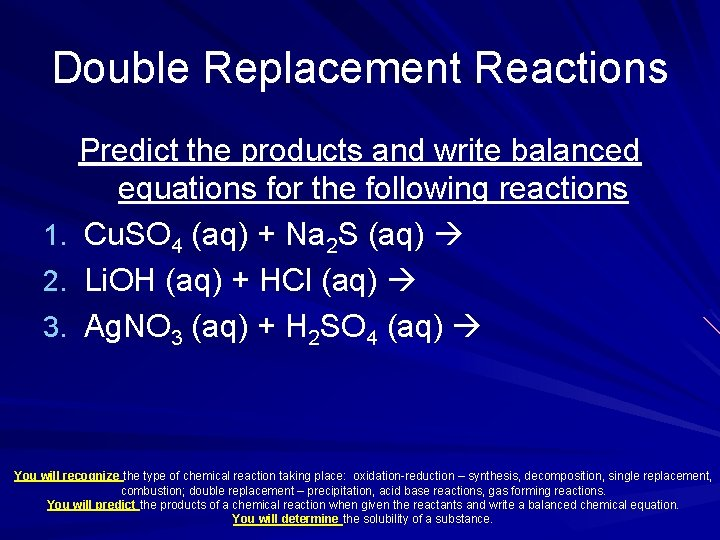Double Replacement Reactions 1. 2. 3. Predict the products and write balanced equations for the following reactions Cu. SO 4 (aq) + Na 2 S (aq) Li. OH (aq) + HCl (aq) Ag. NO 3 (aq) + H 2 SO 4 (aq) You will recognize the type of chemical reaction taking place: oxidation-reduction – synthesis, decomposition, single replacement, combustion; double replacement – precipitation, acid base reactions, gas forming reactions. You will predict the products of a chemical reaction when given the reactants and write a balanced chemical equation. You will determine the solubility of a substance.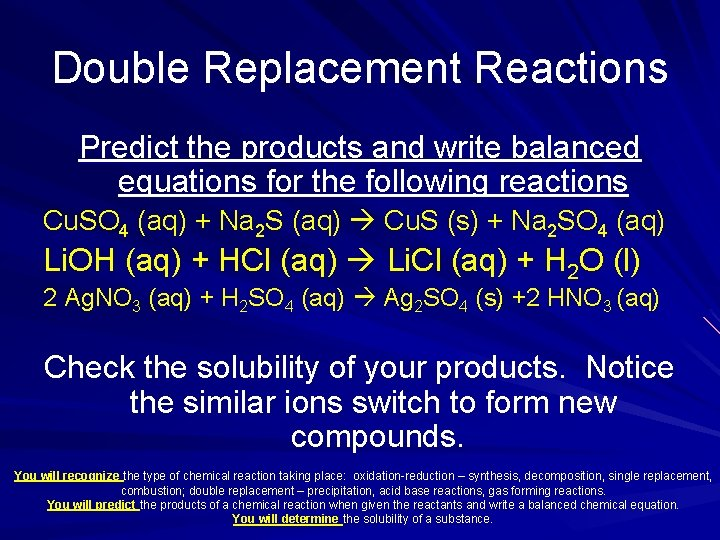Double Replacement Reactions Predict the products and write balanced equations for the following reactions Cu. SO 4 (aq) + Na 2 S (aq) Cu. S (s) + Na 2 SO 4 (aq) Li. OH (aq) + HCl (aq) Li. Cl (aq) + H 2 O (l) 2 Ag. NO 3 (aq) + H 2 SO 4 (aq) Ag 2 SO 4 (s) +2 HNO 3 (aq) Check the solubility of your products. Notice the similar ions switch to form new compounds. You will recognize the type of chemical reaction taking place: oxidation-reduction – synthesis, decomposition, single replacement, combustion; double replacement – precipitation, acid base reactions, gas forming reactions. You will predict the products of a chemical reaction when given the reactants and write a balanced chemical equation. You will determine the solubility of a substance.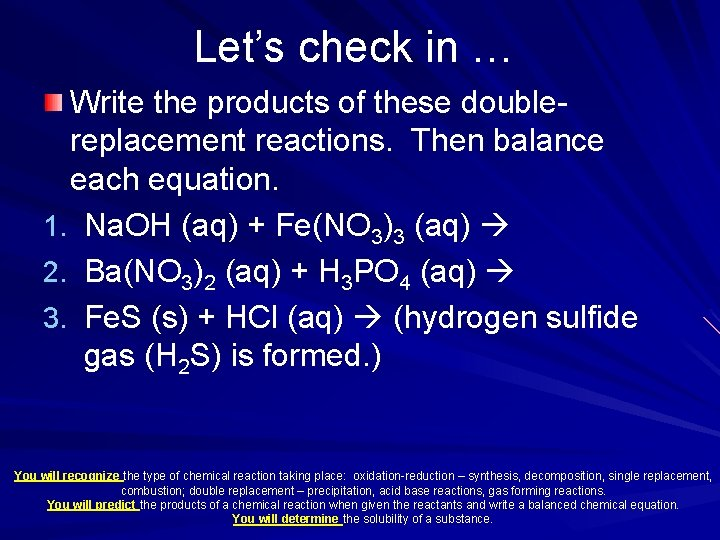Let’s check in … Write the products of these doublereplacement reactions. Then balance each equation. 1. Na. OH (aq) + Fe(NO 3)3 (aq) 2. Ba(NO 3)2 (aq) + H 3 PO 4 (aq) 3. Fe. S (s) + HCl (aq) (hydrogen sulfide gas (H 2 S) is formed. ) You will recognize the type of chemical reaction taking place: oxidation-reduction – synthesis, decomposition, single replacement, combustion; double replacement – precipitation, acid base reactions, gas forming reactions. You will predict the products of a chemical reaction when given the reactants and write a balanced chemical equation. You will determine the solubility of a substance.How did you do? 1. 3 Na. OH (aq) + Fe(NO 3)3 (aq) Fe(OH)3 (s) + 3 Na. NO 3 (aq) 2. 3 Ba(NO 3)2 (aq) + 2 H 3 PO 4 (aq) Ba 3(PO 4)2 (s) + 6 HNO 3 (aq) 3. Fe. S (s) + 2 HCl (aq) Fe. Cl 2 (s) + H 2 S (g) You will recognize the type of chemical reaction taking place: oxidation-reduction – synthesis, decomposition, single replacement, combustion; double replacement – precipitation, acid base reactions, gas forming reactions. You will predict the products of a chemical reaction when given the reactants and write a balanced chemical equation. You will determine the solubility of a substance.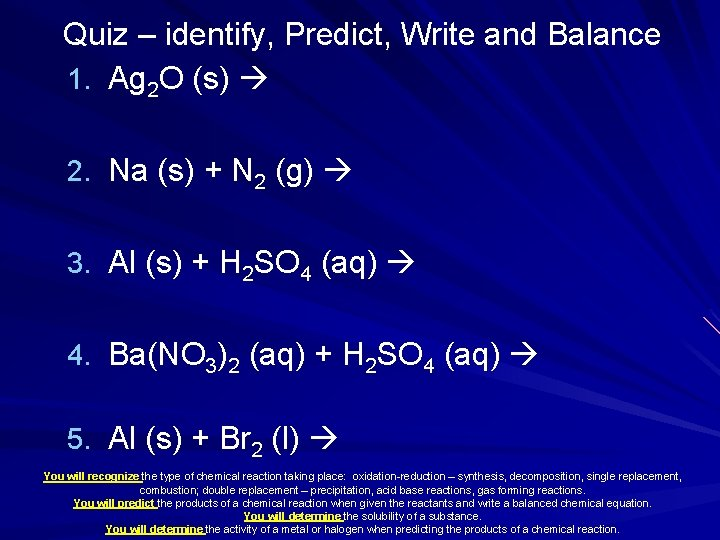Quiz – identify, Predict, Write and Balance 1. Ag 2 O (s) 2. Na (s) + N 2 (g) 3. Al (s) + H 2 SO 4 (aq) 4. Ba(NO 3)2 (aq) + H 2 SO 4 (aq) 5. Al (s) + Br 2 (l) You will recognize the type of chemical reaction taking place: oxidation-reduction – synthesis, decomposition, single replacement, combustion; double replacement – precipitation, acid base reactions, gas forming reactions. You will predict the products of a chemical reaction when given the reactants and write a balanced chemical equation. You will determine the solubility of a substance. You will determine the activity of a metal or halogen when predicting the products of a chemical reaction.Quiz Answers, 1 -5 1. 2 Ag 2 O (s) 4 Ag + O 2 (decomposition) 2. 6 Na (s) + N 2 (g) 2 Na 3 N (synthesis) 3. 2 Al (s) + 3 H 2 SO 4 (aq) Al 2(SO 4)3 + 3 H 2 (single replacement) 4. Ba(NO 3)2 (aq) + H 2 SO 4 (aq) Ba. SO 4 (s) + 2 HNO 3 (aq) (double replacement) 5. 2 Al (s) + 3 Br 2 (l) 2 Al. Br 3 (synthesis) You will recognize the type of chemical reaction taking place: oxidation-reduction – synthesis, decomposition, single replacement, combustion; double replacement – precipitation, acid base reactions, gas forming reactions. You will predict the products of a chemical reaction when given the reactants and write a balanced chemical equation. You will determine the solubility of a substance. You will determine the activity of a metal or halogen when predicting the products of a chemical reaction.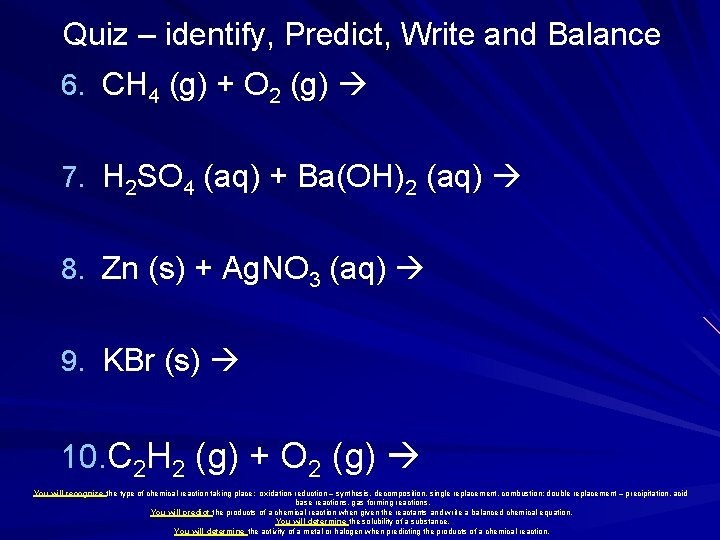Quiz – identify, Predict, Write and Balance 6. CH 4 (g) + O 2 (g) 7. H 2 SO 4 (aq) + Ba(OH)2 (aq) 8. Zn (s) + Ag. NO 3 (aq) 9. KBr (s) 10. C 2 H 2 (g) + O 2 (g) You will recognize the type of chemical reaction taking place: oxidation-reduction – synthesis, decomposition, single replacement, combustion; double replacement – precipitation, acid base reactions, gas forming reactions. You will predict the products of a chemical reaction when given the reactants and write a balanced chemical equation. You will determine the solubility of a substance. You will determine the activity of a metal or halogen when predicting the products of a chemical reaction.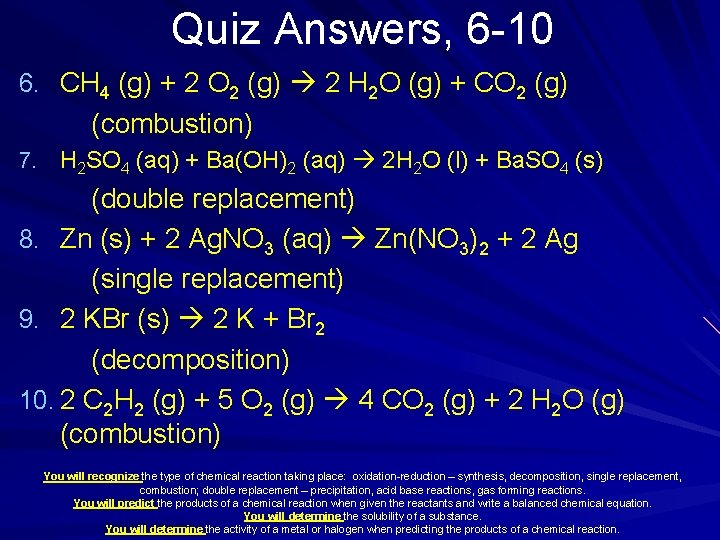Quiz Answers, 6 -10 6. CH 4 (g) + 2 O 2 (g) 2 H 2 O (g) + CO 2 (g) (combustion) 7. H 2 SO 4 (aq) + Ba(OH)2 (aq) 2 H 2 O (l) + Ba. SO 4 (s) (double replacement) 8. Zn (s) + 2 Ag. NO 3 (aq) Zn(NO 3)2 + 2 Ag (single replacement) 9. 2 KBr (s) 2 K + Br 2 (decomposition) 10. 2 C 2 H 2 (g) + 5 O 2 (g) 4 CO 2 (g) + 2 H 2 O (g) (combustion) You will recognize the type of chemical reaction taking place: oxidation-reduction – synthesis, decomposition, single replacement, combustion; double replacement – precipitation, acid base reactions, gas forming reactions. You will predict the products of a chemical reaction when given the reactants and write a balanced chemical equation. You will determine the solubility of a substance. You will determine the activity of a metal or halogen when predicting the products of a chemical reaction.